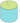# Cylinder Volume and Area Calculator

Height

Select Unit### Calculate Cylinder Volume?

The Volume of a right circular cylinder is: Volume = π * radius2 * height .
ex ; Volume = π * 102 * 20 = 6283.19 m3

### How to get height of Circular Cylinder?

height Cylinder = Volume/π * radius2 .

### Get the radius of Cylinder :

Radius of Cylinder = √Volume / π * height .

### Cylinder area ?

#### The Lateral Area of a right circular cylinder :

lateral area = 2 * π * radius * height .
ex : Lateral area = 2 * π * 10 * 20 = 1256.64 m2

#### The Surface Area of a right circular cylinder :

Surface area = ( 2 * π * radius * height) + ( 2 * π * radius2)

#### The Base Area or Top area of circular cylinder :

Base area = π * radius2 .
Top area = π * radius2 .